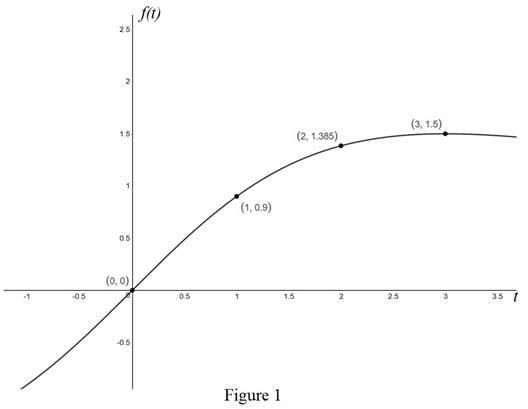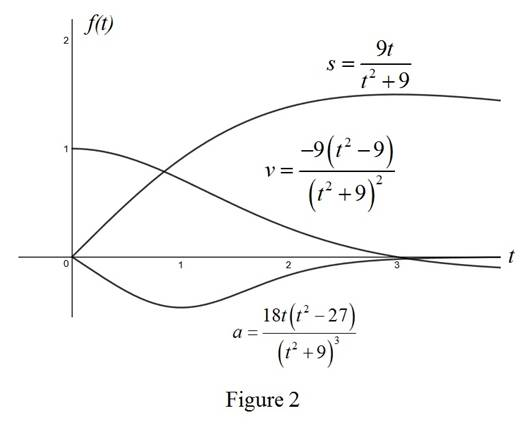# The velocity at time t .### Single Variable Calculus: Concepts...

4th Edition
James Stewart
Publisher: Cengage Learning
ISBN: 9781337687805### Single Variable Calculus: Concepts...

4th Edition
James Stewart
Publisher: Cengage Learning
ISBN: 9781337687805

#### Solutions

Chapter 3.8, Problem 2E

(a)

To determine

## To find: The velocity at time t.

Expert Solution

The velocity at time t is f'(t)=9(t29)(t2+9)2ft/sec_.

### Explanation of Solution

Given:

The given equation is as below.

s=f(t)=9tt2+9 (1)

Calculation:

Calculate the velocity at time t.

Differentiate the equation (1) with respect to time by applying uv method.

f'(t)=(t2+9)(9)(9t×(2t))(t2+9)2=9t2+8118t2(t2+9)2=9t2+81(t2+9)2

f'(t)=9(t29)(t2+9)2 (2)

Therefore, the velocity at time t is f'(t)=9(t29)(t2+9)2ft/sec_.

(b)

To determine

### To find: The velocity after 1 second.

Expert Solution

The velocity after 1 second is v(1)=0.72ft/sec_.

### Explanation of Solution

Calculate the velocity after 1 second.

Substitute 1 for t in the equation (2).

v(t)=f'(t)=9(t29)(t2+9)2v(1)=9(19)(1+9)2=0.72ft/sec

Therefore, the velocity after 1 second is v(1)=0.72ft/sec_.

(c)

To determine

### To find: The time when particle at rest.

Expert Solution

The time when particle at rest is t=3sec_.

### Explanation of Solution

Calculate the time when particle at rest.

The velocity will be zero when the particle is at rest.

Substitute 0 for v(t) in the equation (2).

v(t)=9(t29)(t2+9)20=9(t29)(t2+9)29(t29)=0t29=0t=3sec

Therefore, the time when particle at rest is t=3sec_.

(d)

To determine

### To find: The particle moving in the positive direction.

Expert Solution

The particle always moves in positive direction when time is within the range 0t<3_.

### Explanation of Solution

Calculate the time during which the particle will be moving in the positive direction.

If the particle moves in positive direction, the velocity at any time t will be greater than zero.

v(t)=9(t29)(t2+9)2>09(t29)(t2+9)2>09(t29)>0t29>0t<3

Therefore, the particle moving in the positive direction when 0t<3_.

(e)

To determine

### To find: The total distance traveled during the first 6 seconds.

Expert Solution

The total distance travelled during first 6 second is 1.8ft_.

### Explanation of Solution

Calculate the total distance traveled during first 6 seconds.

Here, the particle moves in positive and negative direction, the total distance traveled should be calculated between the intervals of [0,3] and [3,6].

Substitute 3 and 0 for t between interval of [0,3] in the equation (1) and subtract them.

|f(3)f(0)|=|9(3)(3)2+99(0)(0)2+9|=|320|=32

Substitute 6 and 3 for t between intervals of [3,6] for t in the equation (1) and subtract them.

|f(6)f(3)|=|9(6)(6)2+99(3)(3)2+9|=|6532|=310

The total distance travelled is as given below.

f(6)=32+310=1.8ft

Therefore, the total distance travelled during first 6 second is f(6)=1.8ft_.

(f)

To determine

Expert Solution

### Explanation of Solution

Show the diagram to illustrate the motion of the particle as shown below in Figure 1.Figure 1 shows the movement of particle at different times.

(g)

To determine

### To find: The acceleration at time t and after 1 second.

Expert Solution

The acceleration at time t is f"(t)=18t(t227)(t3+9)3_ and after one second is 0.468ft/s2_.

### Explanation of Solution

Calculate the acceleration at time t.

Differentiate the equation (2) with respect to t by applying uv method.

f'(t)=9(t29)(t2+9)2f"(t)=9(t2+9)2(2t)[(t29)(2(t2+9)×2t)][(t2+9)2]2=92t(t2+9)[(t2+9)2(t29)](t2+9)4

On further simplification,

f"(t)=18t[t2+92t2+18](t2+9)3=18t(t2+27)(t2+9)3=18t(t227)(t3+9)3

Therefore, the acceleration at time is f"(t)=18t(t227)(t3+9)3_.

Calculate the acceleration after 1 second.

Substitute 1 for t in the above equation.

f"(t)=18t(t227)(t3+9)3f"(1)=18(1)((1)227)(13+9)3=0.468fts2

Therefore, the acceleration after 1 second is f"(1)=0.468fts2_.

(h)

To determine

Expert Solution

### Explanation of Solution

Calculate the position of the particle with respect to time using the expression.

s(t)=9tt2+9

Substitute 0 for t in the above equation.

s(0)=9(0)02+9=0

Similarly, calculate the remaining values.

Calculate the value of t and s(t) as shown in the table (1).

 t s(t)=9tt2+9 0 0 0.5 0.48649 1 0.9 1.5 1.2 2 1.38462 2.5 1.47541 3 1.5 3.5 1.48235 4 1.44 4.5 1.38462 5 1.32353 5.5 1.26115 6 1.2

Calculate the velocity using the formula.

v(t)=9(t29)(t2+9)2

Substitute 0 for t in the above equation.

v(0)=9(029)(02+9)2=1

Similarly, calculate the remaining values.

Calculate the value of t and v(t) as shown in the table (1).

 t v(t)=−9(t2−9)(t2+9)2 0 1 0.5 0.92038 1 0.72 1.5 0.48 2 0.26627 2.5 0.10642 3 0 3.5 -0.0648 4 -0.1008 4.5 -0.1183 5 -0.1246 5.5 -0.1241 6 -0.12

Calculate the acceleration using the formula.

a(t)=18t(t227)(t3+9)3

Substitute 0 for t in the above equation.

a(0)=18(0)(0227)(03+9)3=0

Similarly, calculate the remaining values.

Calculate the value of t and a(t) as shown in the table (1).

 t a(t)=18t(t2−27)(t3+9)3 0 0.00 0.5 -0.32 1 -0.47 1.5 -0.35 2 -0.17 2.5 -0.06 3 -0.02 3.5 -0.01 4 0.00 4.5 0.00 5 0.00 5.5 0.00 6 0.00

Draw the graph of the position, velocity and acceleration functions as shown in the Figure 2.(i)

To determine

### To find: The time when particle speeding up and slowing down.

Expert Solution

the acceleration is zero when time t is greater than 33sec and the acceleration is negative when time t is less than 33sec.

### Explanation of Solution

Calculate the time when particle is speeding up and slowing down.

Substitute 0 for f"(t) in the equation f"(t)=18t(t227)(t3+9)3.

0=18t(t227)(t3+9)3t227=0t=27t=33sec

Substitute 1sec for t in the equation f"(t)=18t(t227)(t3+9)3.

f"(1)=18×(1)((1)227)((1)3+9)3=0.468<0

Substitute 4sec for t in the equation f"(t)=18t(t227)(t3+9)3.

f"(4)=18×(4)((4)227)((4)3+9)3=0

Therefore, the acceleration is zero when time t is greater than 33sec and the acceleration is negative when time t is less than 33sec.

### Have a homework question?

Subscribe to bartleby learn! Ask subject matter experts 30 homework questions each month. Plus, you’ll have access to millions of step-by-step textbook answers!